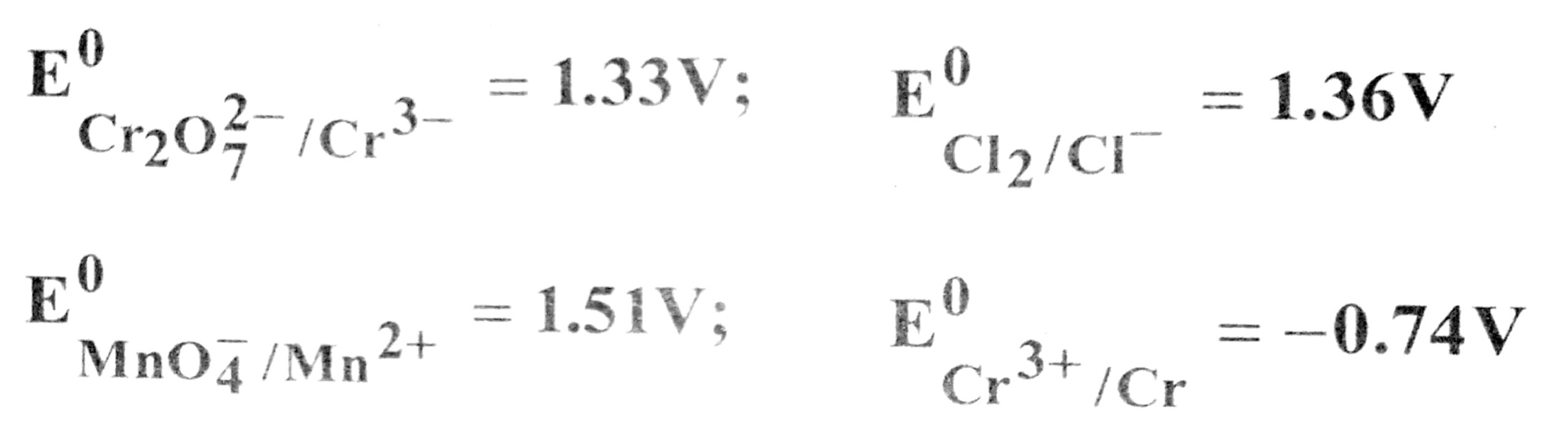3. ELECTROCHEMISTRY

1. If 96500 coulomb electricity is passed through CusO4 solution, it will liberate

(A) 63.5 gm of Cu
(B) 31.76 gm of Cu
(C) 96500 gm of Cu
(D) 100 gm of Cu

2. Fused NaCl on electrolysis gives …………… on cathode.

(A) Chlroine
(B) Sodium
(C) Sodium amalgam
(D) Hydrogen

3. The standard electrode potentials for the half cell reactions are :

Zn → Zn2+ + 2e- E° = + 0.76 V
Fe → Fe2+ + 2e- E° = + 0.41 V
The emf of the cell reaction Fe2+ + Zn → Zn2+ + Fe is

(A) – 0.35 V
(B) + 0.35 V
(C) – 1.17 V
(D) + 1.17 V

4. For a certain redox reaction, Eo is positive. This means that

(A) ΔG° is positive, K is greater than 1
(B) ΔG° is positive, K is less than 1
(C) ΔG° is negative, K is greater than 1
(D) ΔG° is negative, K is less than 1

5.Cell reaction is spontaneous, when

(A) E0red is negative
(B) ΔG° is negative
(C) E0oxid is positive
(D) ΔG° is positive

6. Equilibrium constant K is related to E0cell and not Ecell because

(A) E0cell is easier to measure than Ecell
(B) Ecell becomes zero at equilibrium point but E0cell remains constant under all conditions
(C) at a given temperature, Ecell changes hence value of K can’t be measured
(D) any of the terms Ecell or E0cell can be used

7. Molar conductivity of 0.15 M solution of  KC1 at 298 K, if its conductivity of 0.0152 S cm-1 will be

(A) 124 Ω-1 cm2 mol-1
(B) 204 Ω-1 cm2 mol-1
(C) 101 Ω-1 cm2 mol-1
(D) 300 Ω-1 cm2 mol-1

8. The specific conductivity of N/10 KCl solution at 20°C is 0.0212 ohm-1 cm-1 and the resistance of the cell containing this solution at 20°C is 55 ohm. The cell constant is

(A) 3.324 cm-1
(B) 1.166 cm-1
(c) 2.372 cm-1
(D) 3.682 cm-1

9. Faraday’s law of electrolysis is related to

(A) Atomic number of cation
(B) Speed of cation
(C) Speed of anion
(D) Equivalent weight of electrolyte

10. The molar conductivity is maximum for the solution of concentration

(A) 0.004 M
(B) 0.002 M
(C) 0.005 M
(D) 0.001 M

11. Units of the properties measured are given below. Which of the properties has been not matched correctly ?

(A) Molar conductance = Sm2 mol-1
(B) Cell constant = m-1
(C) Specific conductance of = S m2
(D) Equivalence conductance = S m2 (g eq)-1

12. How long would it take to deposit 50 g of Al from an electrolytic cell containing Al2O3 using a current of 105 ampere ?

(A) 1.54 h
(B) 1.42 h
(C) 1.32 h
(D) 2.15 h

13. The charge required for reducing 1 mole of MnOto Mn2+ is

(A) 1.93 x 105 C
(B) 2.895 x 105 C
(C) 4.28 x 105 C
(D) 4.825 x 105 C

14. If a current of 1.5 ampere flows through a metallic wire for 3 hours, then how many electrons would flow through the wire ?

(A) 2.25 x 1022 electrons
(B) 1.13 x 1023 electrons
(C) 1.01 X 1023 electrons
(D) 4.5 x 1023 electrons

15. How many coulombs of electricity is required to reduce 1 mole of Cr2Oin acidic medium ?

(A) 4 96500 C
(B) 6 x 96500 C
(C) 2 x 96500 C
(D) 1 x 96500 C

16. How much time is required to deposit 1 X 10-3 cm thick layer of silver (density of 1.05 g cm-3) on a surface of area 100 cm2 by passing a current of 5 A through AgNO3 solution ?

(A) 125 s
(B) 115 s
(C) 18.7 s
(D) 27.25 s

17. How much metal will be deposited when a current on 12 ampere with 75% efficiency is passed through the cell for 3 h ? (Given : Z = 4 x 10-4)

(A) 32.4 g
(B) 38.8 g
(C) 36.0 g
(D) 22.4 g

18. An electrochemical cell can behave like an electrolytic cell when

(A) Ecell = 0
(B) Ecell > Eext
(C) Eext > Ecell
(D) Ecell = Eext

19. Use the data given below find out the strongest reducing agent.(A) Cl
(B) Mn2+
(C) MnO(D) Cr3+

20. The quantity of charge required to obtain one mole of aluminium from A12O3 is

(A) 1 F
(B) 6 F
(C) 3 F
(D) 2 F

21. The cell constant of a conductivity cell

(A) changes with change of electrolyte
(B) changes with change of concentration of electrolyte
(C) changes with temperature of electrolyte
(D) remains constant for a cell

22. While charging the lead storage battery

(A) PbSO4 anode is reduced to Pb
(B) PbSO4 cathode is reduced to Pb
(C) PbSO4 cathode is oxidised to Pb
(D) PbSO4 anode is oxidised to Pbo2

23. In the electrolysis of aqueous sodium chloride solution which of the half cell reaction will occur at anode ?

(A) Na+(aq) +e → Na(s); E0cell = -2.71V
(B) 2H2IO(l) → O2 (g) + 4H+(aq) + 4e;E0cell = 1.23V
(C) H+(aq) + e → 1/2 H2 (g); E0cell = 0.00V
(D) Cl(aq) → 1/2 Cl2 (g)+e-;E0cell = 1.36V

24. When one faraday of electric current is passed, the mass deposited, is equal to

(A) One gram equivalent
(B) One gram mole
(C) electrochemical equivalent
(D) Half gram equivalent

25. The electrode through which electrons enter into electrolytic solution is

(A) Cathode
(B) Anode
(C) May be anode cathode
(D) None